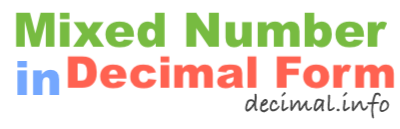What is 7 1/8 in decimal form?To start off, note that 7 1/8 is a mixed number, also known as a mixed fraction. It has a whole number and a fractional number. We have labeled the parts of the mixed number below so it is easier to follow along.

7 = Whole number
1 = Numerator
8 = Denominator

To get 7 1/8 in decimal form, we basically convert the mixed number to a fraction and then we divide the numerator of the fraction by the denominator of the fraction. Here are the detailed math steps we use to convert 7 1/8 mixed number to decimal form:

Step 1: Multiply the whole number by the denominator:

7 × 8 = 56

Step 2: Add the product you got in Step 1 to the numerator:

56 + 1 = 57

Step 3: Divide the sum from Step 2 by the denominator:

57 ÷ 8 = 7.125

That's it folks! The answer to 7 1/8 in decimal form is displayed below:

7 1/8 ≈ 7.13

Mixed Number in Decimal Form
7 1/8 in decimal form is not all we can do! Here you can convert another mixed number to decimal form.

What is 7 1/9 in decimal form?
Here is the next mixed number on our list that we have converted into decimal form.